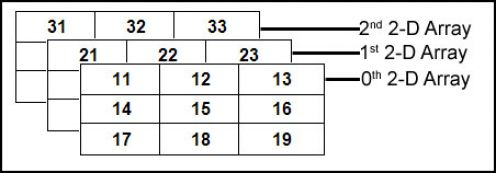# Applications of Array

In this article, we are going to go through the application of an important data structure of any programming language. We are talking about arrays.

An array is a linear data structure which is used to store collection of similar type of data each of which can be accessed by using an index. The memory location of every element can be calculated by using a simple mathematical formula. The main advantage of an array over any other data structure is any location can be accessed in constant time in an array which makes it so useful in the design of other data structures. So an array is used as a basic building block in many data structures.

## Applications of Array• Arrays are used to implement vectors and lists which are an important part of C++ STL.
• Arrays are also used to implement stack and queues.
• Trees also use array implementation whenever possible as arrays are easy to handle compared to pointers. Tress, in turn, are used to implement various other types of data structures.
• Matrices which are an important part of the mathematical library in any programming languages is implemented using arrays.
• Adjacency list implementation of graph uses vectors which are again implemented using arrays.
• Data structures like a heap, map, and set use binary search tree and balanced binary trees which uses can be implemented using arrays.
• Arrays are used to maintain multiple variables with the same name.
• CPU scheduling algorithms use implemented using arrays.
• All sorting algorithms use arrays at its core.

Other than these mentioned above there are tons of uses of arrays. Any modern code CANNOT be implemented without arrays.